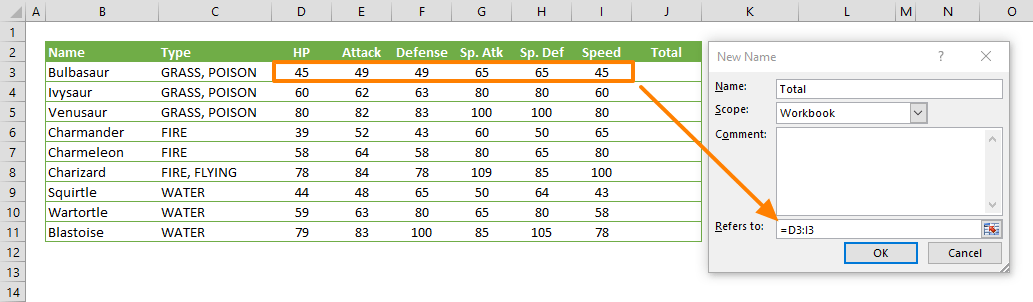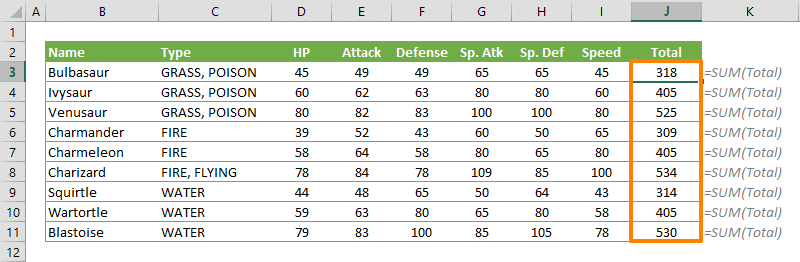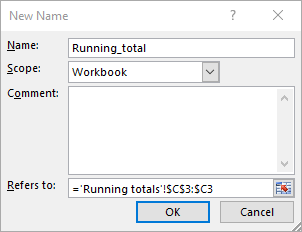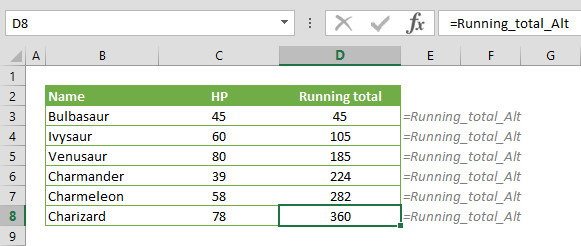Excel uses absolute references for named ranges by default. While absolute references can cover most scenarios, relative references in named ranges work as relative references do in formulas. This behavior can be useful in some scenarios, but can be a bit tricky! In this guide, we're going to show you how to use relative named ranges in Excel formulas.

Relative references in named ranges change when the formula is moved or copied to another cell. Let's see how this works on an example.

Here, we have a table which contains numerical data between the D and I columns. When cell J3 is selected, opening the New Name dialog allows editing the reference of the named range.

The name of this new named range is "Total" and for the Refers to field, we selected the range D3:I3 as a relative reference.If you are not familiar with creating named ranges, please visit our guide 5 Ways to Create an Excel Named Range

A formula like the following returns the sum the values in the range C3:I3.

=SUM(Total)

This statement is correct only when the formula is entered into cell J3. For other cells, the named range represents a range that starts 5 columns before and ends 1 column before.

In the following screenshot, you can see that cells under column J have the same formula. However, all formulas return different values relative to their position.## Alternative uses of relative named ranges

### Handling dynamic data

Inserting a new row to the end of a data set is a common scenario, but formulas will not be updated when you do so.You can overcome this by using a relative named range instead. Here are the steps:

1. Select the cell (i.e. B1)
2. Open the New Name dialog
3. Set a friendly name (i.e. "LastCell")
4. Enter =A1 to the Refers to field
5. Create the named rangeAfter creating the named range, activate the cell that contains the SUM function and use the named range in range notation.

=SUM(D3:LastCell)Since the reference of the named range "LastCell" will be updated after moving the formula, you will always get the correct value.

### Running total calculation

Actually this is a mixed references trick. However, we can use relative named ranges as well. Here is how:

1. Select the cell where running totals will start
2. Open the New Name dialog
3. Give a friendly name (i.e. "Running_total")
4. Select the cell where data starts (C3 in our example)
5. Excel writes the cell with absolute references. It's OK!
6. Type in ":" and the same cell without the \$ characters (i.e. ='Running totals'!\$C\$3:\$C3)
7. Save the nameAfter creating the named range all you need to is use the named range in the SUM formula.Alternatively, you can place the SUM function into the named range. However, remember to select the cell D3 before any updates in the named range.With this named range, users can only see the name.